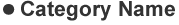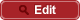• HOME
• RIKEN-QHP
• Measurement of $\eta_c(1S)$, $\eta_c(2S)$ and non-resonant $\eta' \pi^+ \pi^-$ production via two-photon collisions

##PrePrint No. RIKEN-QHP-394 Q. N. Xu et al., (Belle Collaboration) including M. Takizawa Measurement of $\eta_c(1S)$, $\eta_c(2S)$ and non-resonant $\eta' \pi^+ \pi^-$ production via two-photon collisions arXiv: 1805.03044   (org) Phys. Rev. D 98, 072001 (2018) 2018.05